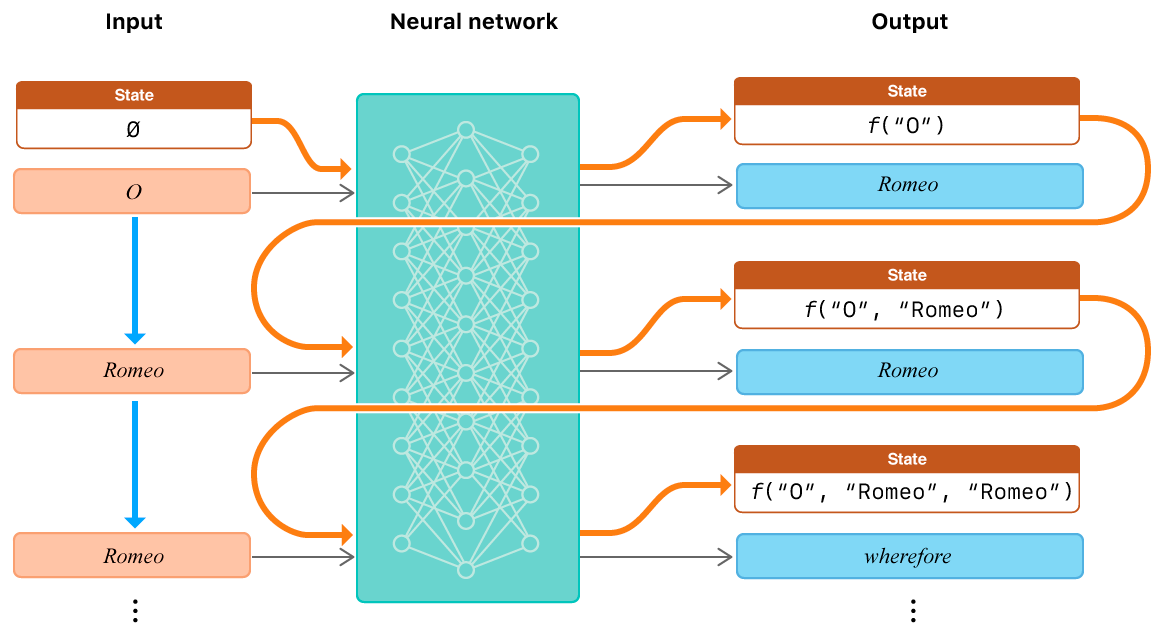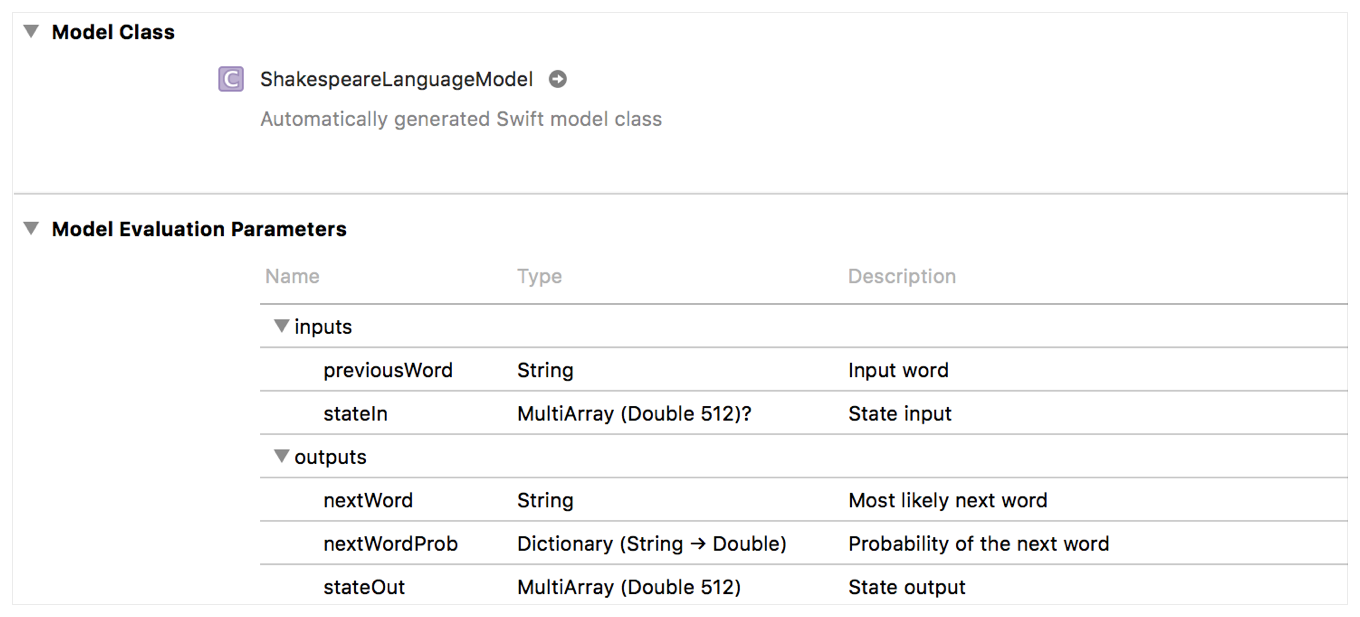Article

# Making Predictions with a Sequence of Inputs

Integrate a recurrent neural network model to process sequences of inputs.

## Overview

Some machine learning problems require more than a single set of inputs and need to process a sequence of inputs over time. Neural network models can process a sequence of inputs, but require some state of the neural network to be maintained between inputs. Core ML provides a straightforward way to maintain the state of the network and process a sequence of inputs.

### Understand the Neural Network Workflow

Processing natural language is a difficult task for machine learning models because the number of possible sentences is infinite, making it impossible to encode all the inputs to the model. A common approach to reducing the number of possible inputs is to use letters or words as the input to the model, instead of processing the entire sentence as a single input. However, the model then needs a way to maintain state to "remember" what letters or words it has been presented previously in the sequence.

Consider a neural network model trained to generate the Shakespeare play Romeo and Juliet. The neural network encodes the relationship between words and their neighboring words, without using explicit rules. In the popular line, "O, Romeo, Romeo, wherefore art thou Romeo?" the word Romeo appears three times, but each occurrence has a different word following it. The model needs a way to differentiate between the uses. Recurrent neural networks are a class of neural networks that address this problem by using the state of the model after processing each word as additional input when processing a word.Figure 1 The inputs and outputs of a recurrent neural network over three input words

Figure 1 shows an example workflow of a network that has learned Romeo and Juliet. To start the phrase, "O" and a `nil` state are provided as input. The next word is predicted, and the network also generates a representation of its state for the input "O", referred to as f("O"). The next input word "Romeo" is combined with the previous state, f("O"), to create the next input. Given that input, the model again outputs "Romeo" with high probability.

The next input word, "Romeo", is identical to the previous input word. However, the state input is different. The state input is now f("O", "Romeo"). The different state allows the network to output the prediction "wherefore" even though the previous input words were identical.

### Expose the State of the Model

Add a recurrent neural network based model to your project in Xcode to see the state of the neural network exposed as input and output features.Figure 2 An example recurrent neural network that generates the text of Romeo and Juliet

Figure 2 shows the view in Xcode for the `ShakespeareLanguageModel` that has a recurrent neural network layer, with its state input and output features listed. Other recurrent neural networks, like Long Short-Term Memory and Gated Recurrent networks, create input and output features automatically.

This network takes two inputs: the input word and the state input, which is optional. The word is a `String` and the state, named `stateIn`, is a one dimensional `MLMultiArray` of 512 `Double` values. The state input is optional because the beginning of a sequence has no prior state.

There are three outputs of the network: the most probable next word, a `Dictionary` of possible next words paired with their probabilities, and a one dimensional `MLMultiArray` of 512 `Double` values, named `stateOut`, that represent the network's state after processing the input.

The `MLMultiArray` output represents the state of the network, which is the level of activation of its internal nodes. In order for the network to "remember" what input sequence has been processed, the previous output state must accompany the next input.

In practice, you may come across layers with default state feature names. For example, Long Short-Term Memory networks will have default state parameters named `lstm_h_in` and `lstm_c_in` for inputs and `lstm_h_out` and `lsth_c_out` for outputs. The "h" refers to the hidden state and the "c" refers to the cell state used by an LSTM network. These output states must be carried over as input states for the network to function properly across the sequence of inputs.

### Start a Sequence of Inputs

This network was trained to generate the rest of a sentence from the play, given two prompt words from a sentence. Begin processing a sequence of inputs with this model by passing in the first word from the prompt and `nil` as the previous state.

In this sample code the `ShakespeareLanguageModelInput` class, generated by Xcode, is used to store the two inputs for the prediction call.

### Make Predictions Based on Previous State

Create an input using the second word from the prompt and the output state from the prediction as the input state. Use that input with the model to generate a prediction for the third word of the sentence.

When you initialize the network with the first two words, the output state needs to be kept to represent the sequence of inputs. The predicted words and probabilities are ignored. They are ignored because the second word (Romeo) comes from the actual text instead of the model's prediction.

However, once the two word prompt has been processed, the output `nextWord` is the most likely third word in the sentence. It will be used as the input word, to generate the fourth word in the sentence. Using the output as input is repeated to generate the rest of the sentence.

Listing 3 repeats the process of using the predicted word and state as the input word and state until the predicted word is `</s>`. This network uses the string `</s>` to signify the end of the sentence.

### Verify the Output and Reset the Input State

At this point, the model has predicted the end of the sentence. The sequence of `nextWord` values represents the model's prediction for the entire sentence. The entire predicted sentence could be presented to the user for verification or compared to the actual text programmatically.

Reset the input context by using `nil` as the input state (as in Listing 1), to start making predictions on a new sentence.

`MLModel`